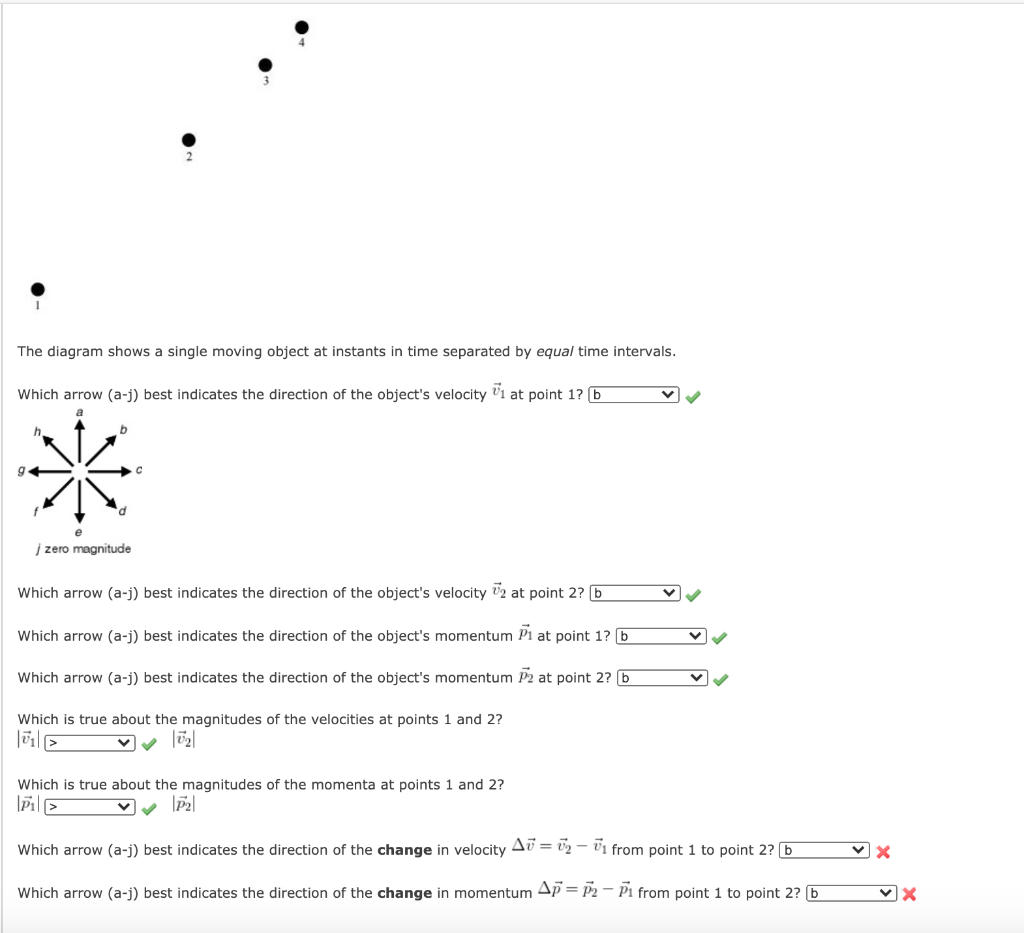# Question Solved1 AnswerThe diagram shows a single moving object at instants in time separated by equal time intervals. Which arrow (a-j) best indicates the direction of the object's velocity 1 at point 1? b * zero magnitude Which arrow (a-j) best indicates the direction of the object's velocity 72 at point 2? b Which arrow (a-j) best indicates the direction of the object's momentum 1 at point 1? b Which arrow (a-j) best indicates the direction of the object's momentum P2 at point 2? b Which is true about the magnitudes of the velocities at points 1 and 2? Which is true about the magnitudes of the momenta at points 1 and 2? ✓ P2 Which arrow (a-j) best indicates the direction of the change in velocity A7 = 72 – Ū from point 1 to point 2? b. Which arrow (a-j) best indicates the direction of the change in momentum Ap = 72 - Đi from point 1 to point 2? b. VXTranscribed Image Text: The diagram shows a single moving object at instants in time separated by equal time intervals. Which arrow (a-j) best indicates the direction of the object's velocity 1 at point 1? b * zero magnitude Which arrow (a-j) best indicates the direction of the object's velocity 72 at point 2? b Which arrow (a-j) best indicates the direction of the object's momentum 1 at point 1? b Which arrow (a-j) best indicates the direction of the object's momentum P2 at point 2? b Which is true about the magnitudes of the velocities at points 1 and 2? Which is true about the magnitudes of the momenta at points 1 and 2? ✓ P2 Which arrow (a-j) best indicates the direction of the change in velocity A7 = 72 – Ū from point 1 to point 2? b. Which arrow (a-j) best indicates the direction of the change in momentum Ap = 72 - Đi from point 1 to point 2? b. VX
More
Transcribed Image Text: The diagram shows a single moving object at instants in time separated by equal time intervals. Which arrow (a-j) best indicates the direction of the object's velocity 1 at point 1? b * zero magnitude Which arrow (a-j) best indicates the direction of the object's velocity 72 at point 2? b Which arrow (a-j) best indicates the direction of the object's momentum 1 at point 1? b Which arrow (a-j) best indicates the direction of the object's momentum P2 at point 2? b Which is true about the magnitudes of the velocities at points 1 and 2? Which is true about the magnitudes of the momenta at points 1 and 2? ✓ P2 Which arrow (a-j) best indicates the direction of the change in velocity A7 = 72 – Ū from point 1 to point 2? b. Which arrow (a-j) best indicates the direction of the change in momentum Ap = 72 - Đi from point 1 to point 2? b. VX